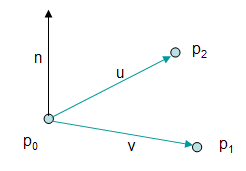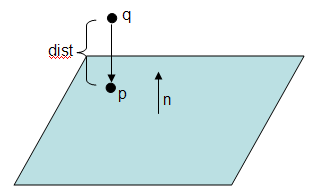Prev: Line and Rays Next: Ray-Sphere Intersection

A 3D plane can be defined in many ways, however all of them can be derived from the simple case where we have three points.

One of the most common ways to define a plane is with the following equation:

`    	Ax + By + Cz + D = 0`

Assuming three points p0, p1, and p2 the coefficients A, B, C and D can be computed as follows:

• Compute vectors v = p1p0, and u = p2p0;
• Compute n = v x u (cross product)
• Normalize n
• Assuming n = (xn,yn,zn) is the normalized normal vector then
• A = xn
• B = yn
• C = zn
• To compute the value of D we just use the equation above, hence -D = Ax + By + Cz. Replacing (x,y,z) for a point in the plane (for instance p0), we get D = – n . p0 (dot product).

The following figure presents all the intervenients in this process.Distance from a point to a plane

Assuming that the equation Ax + By + Cz + D = 0 has been obtained as shown above then the distance from the plane to a point r(rx,ry,rz) can be obtained just by computing the left side of equation, or

`    dist = A*rx + B*ry + C*rz + D = n . r  + D`

Actually the distance is the absolute value of dist, but the sign of dist (if it is zero is on the plane) also gives information as to which side of the plane is point r. If the sign is positive then the point is on the side that agrees with the normal n, otherwise it is on the other side.

A curious fact is that when we consider the distance from the plane to the origin, the distance is D.

Projecting a point to a plane

The projection of a point q on a plane defined by Ax + By + Cz + D = 0 is the point on the plane that is closest to q.

Assume that dist is the signed distance from q to the plane. Then the closest point p on the plane is:

`    p = q - dist * n`

 Prev: Line and Rays Next: Ray-Sphere Intersection

### 2 Responses to “Plane”

1.Am I correct in assuming:
dist = A*rx + A*ry + A*rz + D = n . r + D
should actually be:
dist = A*rx + B*ry + C*rz + D = n . r + D
?

•More than right! What a bug!!!

This site uses Akismet to reduce spam. Learn how your comment data is processed.